## LetsPlayMaths.Com

WELCOME TO THE WORLD OF MATHEMATICS

# Class 3 Length

Length

Conversion of Meters into Centimeters

Conversion of Centimeters into Meters

Conversion of Kilometers into Meters

Conversion of Meters into Kilometers

Addition of Meters and Centimeters Without Carrying

Addition of Meters and Centimeters With Carrying

Subtraction of Meters and Centimeters Without Borrowing

Subtraction of Meters and Centimeters With Borrowing

Multiplication of Meters and Centimeters

Multiplication of Kilometers and Meters

Division of Meters and Centimeters

Division of Kilometers and Meters

Length Test

Length Worksheet

## Length

It is a form of measurement, where we can tell the length of various things like length of a pencil, length of a rope, length of a ship and distance between two cities. In todays world Centimeters, Meters and Kilometers are used as standard units for measurement. To measure smaller lengths like length of a pen, we use centimeters (cm). For measuring length of garden or swimming pool, we use meter (m). For measuring greater lengths as distance between two cities, we use kilometers.

## Conversion of Meters into Centimeters

1 Meter = 100 Centimeters

a) To convert meters into centimeters, multiply the number by 100.

b) To convert meters and centimeters into centimeters, multiply the number of meters by 100 and add

the number of centimeters to it.

Example 1. Convert 65 meters into centimeters

Solution. 65 X 100 = 6500 cm
So, 65 meters is equal to 6500 centimeters

Example 2. Convert 17 m 45 cm into centimeters.

Solution. First, we need to convert 17 meters into centimeters

17 meters = 17 X 100 = 1700 centimeters

Now, add 1700 centimeters with 45 centimeters 1700 cm + 45 cm = 1745 cm

So, 17 m 45 cm is equal to 1745 centimeters

## Conversion of Centimeters into Meters

100 cm = 1 m

Example 1. Convert 475 centimeters into meters

Solution. 475 cm = 400 cm + 75 cm = 4 m 75 cm
So, 475 centimeters is equal to 4 m 75 cm

Example 2. Convert 4365 centimeters into meters

Solution. 4365 cm = 4300 cm + 65 cm = 43 m 65 cm
So, 4365 centimeters is equal to 43 m 65 cm

## Conversion of Kilometers into Meters

1 km = 1000 meters
a) To convert kilometers into meters, multiply the number of kilometers by 1000.

b) To convert the kilometers and meters into meters, multiply the number of kilometers by 1000 and

then add the number of meters.

Example 1. Convert 11 kilometer into meters

Solution. 11 km = 11 X 1000 = 11000 m
So, 11 km is equal to 11000 meters

Example 2. Convert 24 km 745 m into meters.

Solution. 24 km 745 meters = 24 X 1000 m +745 m = 24000 m + 745m = 24745 m
So, 24 km 745 meters is equal to 24745 meters

## Conversion of Meters into Kilometers

1000 meters = 1 kilometers

Example 1. Convert 6000 meters into kilometers

Solution. 6000 m = 6 X 1000 m = 6 X 1 km = 6 km
So, 6000 meters is equal to 6 km

Example 2. Convert 12545 meters into kilometers and meters.

Solution. 12545 m = 12000 m + 545 m = 12 X 1000 m + 545 m = 12 X 1 km + 545 m
= 12 km 545 m

## Addition of Meters and Centimeters Without Carrying

Example 1. Add 45 m 25 cm and 14 m 23 cm

Solution. Arrange the numbers in tabular format as shown below.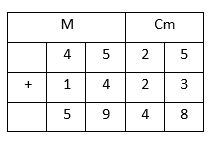Step 1. Add centimeters to centimeters first

25 cm + 23 cm = 48 cm

Write 48 cm under centimeter column

Step 2. Add the meters to meters

45 m + 14 m = 59 m

Write 59 m under meter column

So, the result is 59 m 48

## Addition of Meters and Centimeters With Carrying

Example 1. Add 25 m 75 cm and 15 m 55 cm

Solution. Arrange the numbers in tabular format as shown below.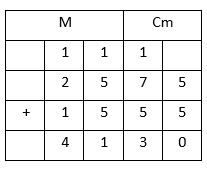Step 1. First add centimeters to centimeters

75 cm + 55 cm = 130 cm = 100 cm + 30 cm = 1 m + 30 cm

Write 30 cm under centimeter column. Carry 1 m to meter column.

Step 2. Add the meters now. 25 m + 15 m + 1 m (Carry over from centimeter addition) = 41 m.

Write 41 m below meter column.

So, the answer is 41 m 30 cm.

## Subtraction of Meters and Centimeters Without Borrowing

Example 1. Subtract 15 m 23 cm from 48 m 55 cm

Solution. Arrange the numbers in tabular format as shown below.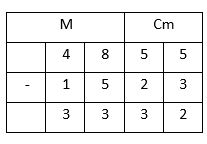Step 1. First subtract centimeters from centimeters.

55 cm  23 cm = 32 cm. Write 32 cm under centimeter column.

Step 2. Subtract meter from meter

48 m  15 m = 33 m. Write 33 m under meter column.

So, the answer is 33 m 32 cm.

## Subtraction of Meters and Centimeters With Borrowing

Example 1. Subtract 15 m 25 cm from 27 m 7 cm.

Solution. Arrange the numbers in tabular format and mention the centimeters in two digits. That is 7 cm
should be written as 07 cm. Here we are following the normal subtraction.So, the answer is 11 m 82 cm.

## Multiplication of Meters and Centimeters

We can multiply meters and centimeters like normal numbers. We must consider two digits centimeters while multiplication. For example, 5m 7cm should be considered as 5m 07 cm. First two digits on the right of the results gives the number of centimeters, the number formed by the remaining digits gives the meters.

Example 1. Multiply 17 m 25 cm by 5.

Solution. Write the numbers in column format as shown below.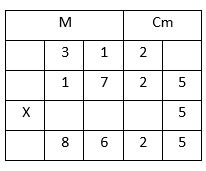So, the answer is 86 m 25 cm.

## Multiplication of Kilometers and Meters

We can multiply kilometers and meters like normal numbers. Meters should always be considered as 3 digits number. For example, 12 km 5 m should be written 12 km 005 m while multiplication.

Example 1. Multiply 24 km 75 m by 8.

Solution.So, the answer is 192 m 600 cm.

## Division of Meters and Centimeters

Division of meters and centimeters can be done by two methods. Both the methods are given below.

Method 1.
Step 1. Convert meters and centimeters into centimeters only.

Step 2. Divide the total number of centimeters by divisor.

Step 3. Convert the quotient (in cm) into meters and centimeters.

Example 1. Divide 9 m 75 cm by 5.

Solution.
Step 1. Convert 9 m 75 cm into centimeters. 9 m + 75 cm = 900 cm + 75 cm = 975 cm

Step 2. Divide 975 cm by 5. 975 ũ 5 = 195 cm

Step 3. Convert 195 cm into meters and centimeters.

195 cm = 100 cm + 95 cm = 1 m 95 cm

So, the answer is 1 m 95 cm

Method 2.

We can divide meters and centimeters like normal division. In this method, centimeters should be of two digits. For example, if the dividend is 24 m 8 cm then it should be written as 24 m 08 cm. when we will bring the first digit of the centimeters down we must write the remaining quotient numbers in the centimeters column.

Example 1. Divide 9 m 5 cm by 5.

Solution. Write the dividend and divisor in the normal division format as shown below.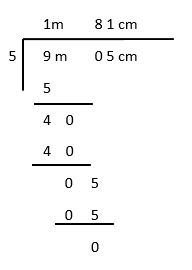## Division of Kilometers and Meters

Division of kilometers and meters can be done by two methods. Both the methods are given below.

Method 1.
Step 1. Convert kilometers and meters into meters only.

Step 3. Divide the total number of meters by divisor.

Step 2. Convert the quotient (in m) into kilometers and meters.

Example 1. Divide 6 km 65 m by 5.

Solution.
Step 1. Convert 6 km 65 m into meters. 6 km + 65 m = 6000 m + 65 m = 6065 m

Step 2. Divide 6065 m by 5. 6065 ũ 5 = 1213 m

Step 3. Convert 1213 m into kilometers and meters.

1213 m = 1000 m + 213 m = 1 km 213 m

So, the answer is 1 km 213 m

Method 2.
We can divide kilometers and meters like normal division. In this method, meters should be of three digits. For example, if the dividend is 24 km 8 m then it should be written as 24 km 008 m. when we will bring the first digit of the meters down we must write the remaining quotient numbers in the meters column.

Example 1. Divide 24 km 5 m by 5.

Solution. Write the dividend and divisor in the normal division format as shown below.So, the answer is 4 km 801 m.

Length Test - 1

Length Test - 2

## Length Worksheet

Length Worksheet - 1